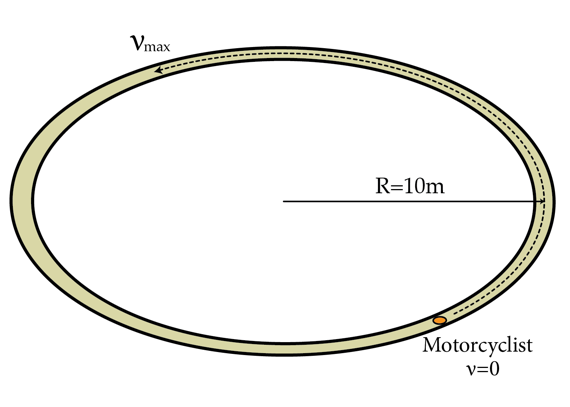# Riding round and round

A motorbike starts from rest at time $t=0$ and begins to accelerate around a circular track as shown in the figure below. Eventually, at time $t=t_1$ the motorbike reaches the maximum velocity possible without slipping off the track. What's the minimum length in meters the motorbike must travel between $t=0$ and $t=t_1$?Details and assumptions

• Assume that the friction coefficient everywhere on the race track is the same.
• The motorbike wheels never slip on the track as it accelerates.
×